Internal Waves 1 - Low frequency

Purpose:

To show the unusual, anisotropic phase and group velocity properties of internal gravity waves in a density-stratified fluid.

What Happens:

A tank about 30 cm deep is filled with a salt stratification of buoyancy period,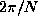, about 6 seconds. A solid cylinder of a few cm diameter runs across the tank at mid depth, in the right of the field of view (see diagram below). This cylinder is oscillated horizontally (to the left and right in the diagram) at frequency less than N, generating internal waves. The flow is visualized with a schlieren system that shows regions of positive isopycnal slope in red, and negative isopycnal slope in green. Slopes close to zero show as yellow. The movie is in time lapse, so that the waves appear to have higher than real frequency. The movie starts from rest, and after the paddle motion begins, the wave field starts to fill the tank outwards from the paddle. In the movie clip, wave energy appears to travel at an anglefrom the horizontal, and the wave crests sweep at right angles to this direction.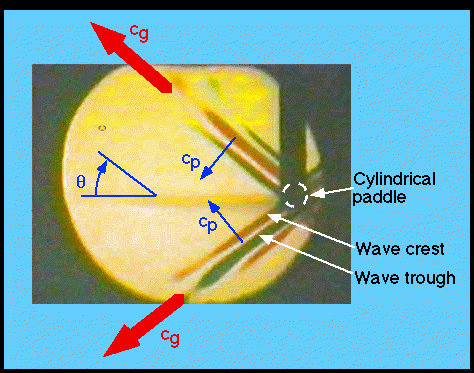Physics of the phenomenon:

a. Dispersion relationship

In a density-stratified fluid at rest, each fluid parcel sits at the level of others of the same density. Lighter parcels sit at a higher level, and denser ones lie below. When the parcel is displaced upwards, it is heavier (more dense) than the surrounding fluid, and the slightly larger gravitational force on that fluid parcel pulls it back down. This is just the same as the settling of a blob of vinegar in a shaken bottle of oil/vinegar salad dressing. The fluid parcel's inertia causes it to overshoot it's starting position, so that when it lies below the rest level, it is lighter (less dense) than its surroundings. The parcel now floats upwards towards its rest level. The fluid parcel therefore bobs up and down, pushed by the buoyancy force generated by gravity acting on slight density differences, and balanced by the inertial forces due to the fluid's mass. The frequency of this oscillation is called the buoyancy frequency, N: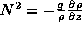where g is the acceleration of the Earth's gravity field, 9.8 ms-2, and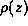is the density of the fluid at rest.

The buoyancy oscillation described above is a special case of an internal gravity wave. If one considers disturbances of the form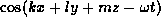, then those disturbances must obey the dispersion relation for internal gravity waves (Phillips, 1966):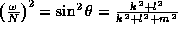This means that the frequency depends on the angle, which is the angle the wave crests and the wave energy flux or group velocity make to the horizontal. Waves of a specific frequency can only propagate at a specific angle. Note also that since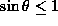, the maximum allowed frequency is N, the frequency of the buoyancy oscillation.

b. Group velocity:

The energy is carried outwards from the paddle by the group velocity, which is restricted to the characteristic angles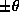, determined by the dispersion relation withpaddle frequency. The wave disturbance builds out from the paddle, but only becomes significant in "ray" regions at the characteristic anglefrom the paddle. Regions between the rays have very little wave energy.

c. Phase velocity

The wave "crests", or lines of constant phase, are the red and green bands. Note that they sweep perpendicular to the energy rays: the phase velocity is perpendicular to the group velocity. Note also the sign: waves above the paddle, with upward moving energy flux, have downward phase propagation, and vice versa. although the phase velocity is the most visible part of the wave propagation, we can never forget that the energy goes with the group velocity, not the phase.

References:

Phillips, O.M. 1966. The dynamics of the upper ocean. Cambridge University Press.

Lighthill, James, 1978. (Chapters 3 and 4.) Waves in fluids. Cambridge University Press.

Credits:

Movie and text - Barry Ruddick
Digitization of movie - Dave Hebert